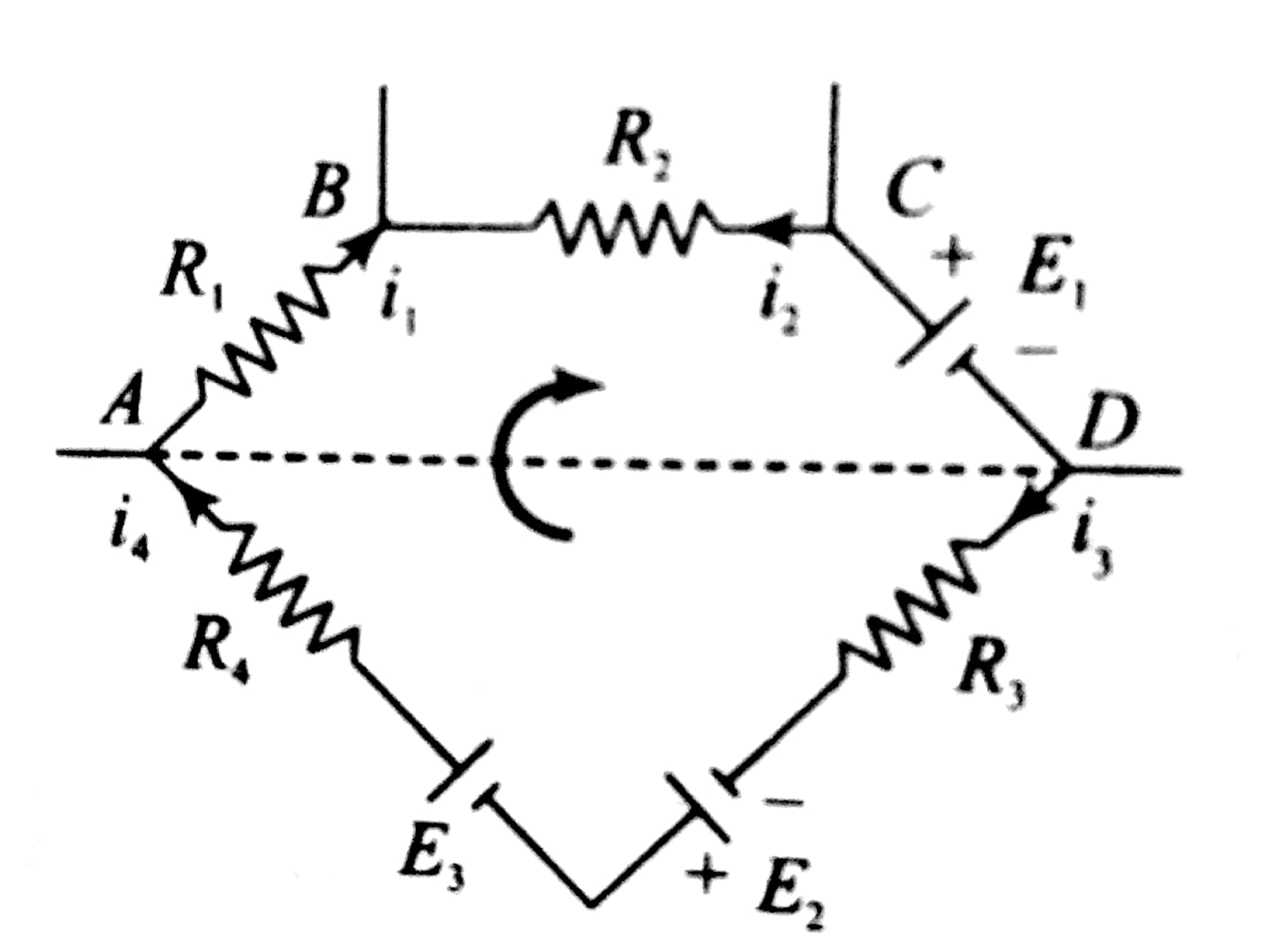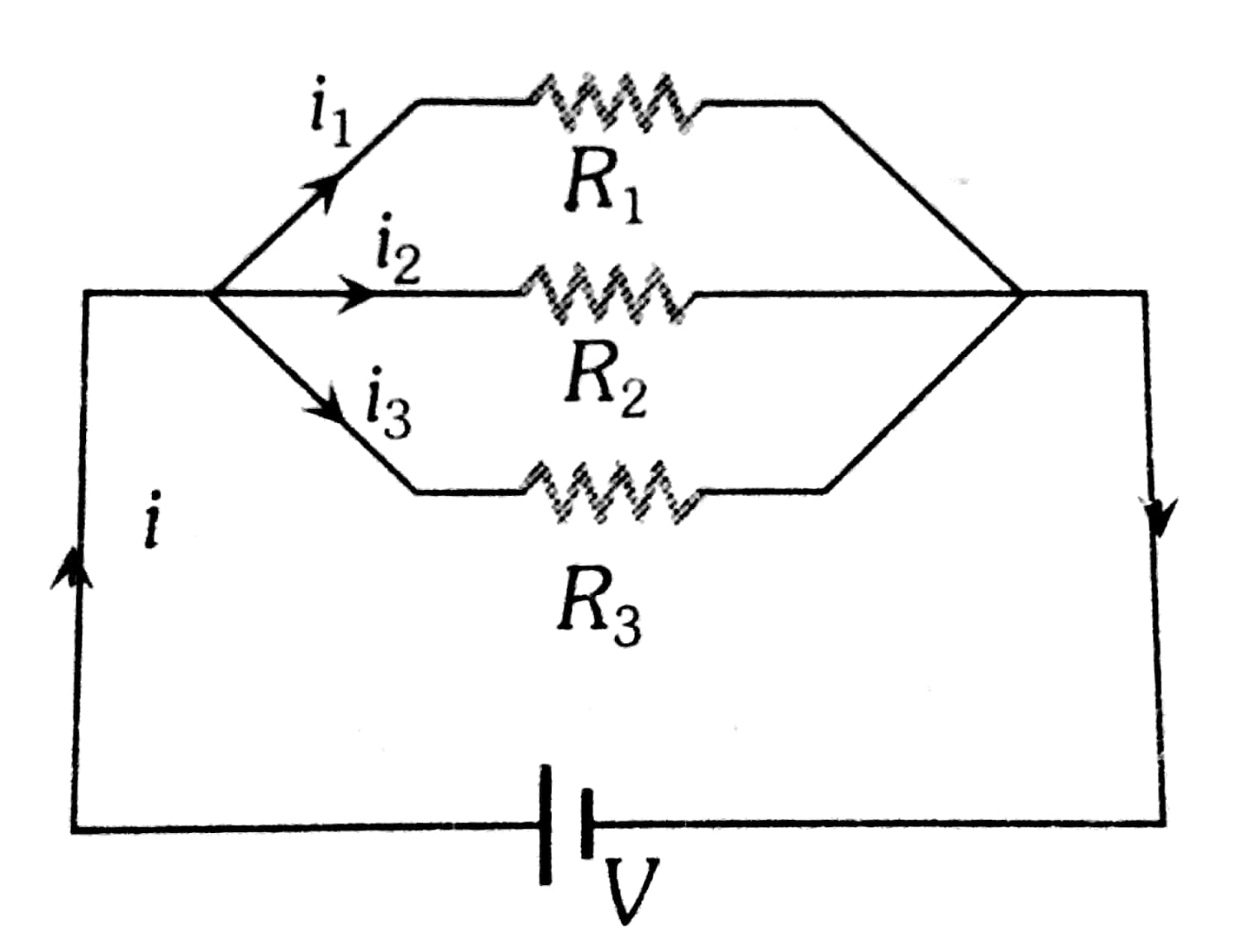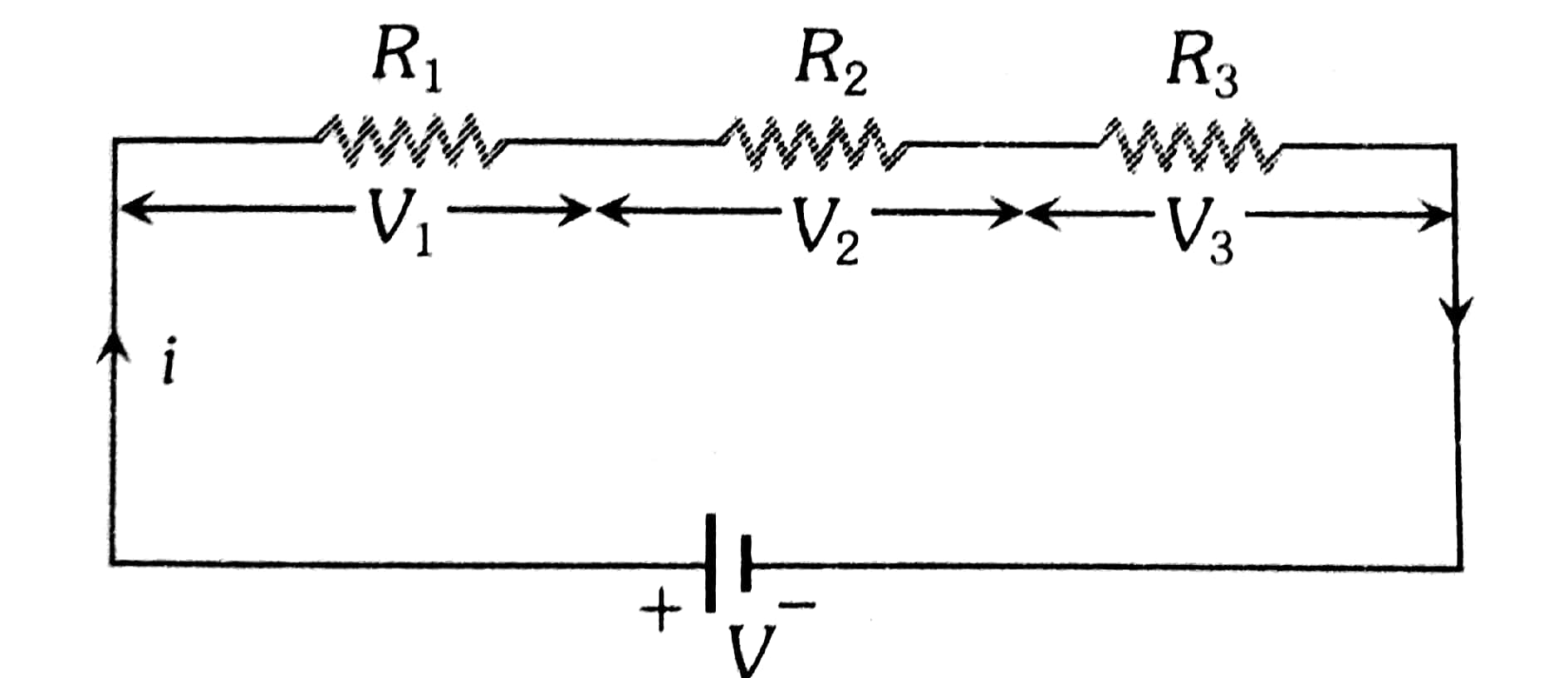## Filters

Sort by :
Clear All
Q
Kirchoff's first law - - wherein   Apply Kcl at Q I6+I3 = I1+ I2   .........(1) I1 = 0.3A I4 = 0.8A I5 = 0.4A KCL at 5 I4= I5 +I3 I3 = I4 - I5     = 0.8 +0.4     =0.4A KCL at R I1+I2 = I4 I2 = I4 - I1     = 0.8 - (-0.3)     =1.1A From equation (1) I6 + I3 = I1+ I2 I6 = I1 + I2 - I3     = -0.3+1.1 -0.4     =0.4A I2 = 1.1A I3 = 0.4A I6 = 0.4A
1. 2T

2. T

3. 3/2T

4. 3T

Radioactive decay,  Where N(t) is the amount of sample which remains after time t. Given that 75% of the sample has decayed, hence the remaining will be 25% of initial,  Also  Take loge both sides and solve Option (1) is correct

What should be the angle between the axes of the polarizer, so that the intensity of the beam coming out of analyzer is 3/8 I

1. 45 degree

2. 30 degree

3. 60 degree

When unpolarized light is passed through the polarizer the intensity becomes half, Hence after passing through polarizer equals  Now after coming out of the analyzer, the intensity becomes  Use Malus Law,  Option (2) is correct.

A potential difference of 1V is applied across the other diagonal. What will be the current in the resistor along the diagonal?

1. 0.5 A

2. 1 A

3. 5 A

4. Zero

By closely observing at the figure, you will find that this circuit is a balanced wheat stone bridge. Hence no current will pass through the diagonal. Option (4) is correct
1. The acceleration is constant both in magnitude and direction.

2. The acceleration is constant in direction but not in magnitude

3. The acceleration is constant in magnitude but not in direction

4. The acceleration is neither constant in magnitude nor in direction.

In any type of motion, Force either changes the direction of particle or magnitude or both. In a uniform circular motion, the velocity of the particle is constant and the magnitude is constant as well and it equals to  . So the direction of the particle changes because of the force, if there was no force then the particle might have followed a straight path rather than a circular path.

So option (3) is correct.

View More

If the speed of the stream is v and the speed of the boat with respect to the stream is u, then which of the following gives the correct relationship between u and v

1. u=v
2. u=3v
3. v=2u
4. v=3u
Velocity of boat w.r.t stream is u  Time taken by boat to go downstream, t = d/ (u+v)  ......(1) Time taken by boat to go upstream, 2t = d/ (u-v)  .........(2) From equation (1) and (2) 2*d/ (u+v) = d/ (u-v) -> 2*(u-v) = (u+v) -> u =3v  Option (2) is correct
represents change enthalpy  And  represents the change in internal energy. According to First law of thermodynamics, H and E are related as,
Engineering
8 Views   |

Question

From a sphere of mass M and radius R, a  smaller sphere of radiusis carved out such that the cavity made in the original sphere is between its centre and the periphery. (See figure). For the configuration in the figure where the distance between the centre of the original sphere and the removed sphere is 3R, the gravitational force between the two spheres is :Question

From a sphere of mass M and radius R, a  smaller sphere of radiusis carved out such that the cavity made in the original sphere is between its centre and the periphery. (See figure). For the configuration in the figure where the distance between the centre of the original sphere and the removed sphere is 3R, the gravitational force between the two spheres is :Solution : Volume of removed sphere, Volume of the sphere (remaining) Therefore mass of sphere carved and remaining sphere are at respectively 1/8M and 7/8M.Therefore, gravitational force between these two sphere,
Engineering
64 Views   |

In a large building, there are 15 bulbs of   40 W, 5 bulbs of 100 W, 5 fans of 80 W and 1 heater of 1 kW.  The voltage of the electric mains is 220 V.  The minimum capacity of the main fuse of the building will be :

• Option 1)

8 A

• Option 2)

10 A

• Option 3)

12 A

• Option 4)

14 A

As we have learned

In parallel Grouping -

-

Resistance of each bulb of 40 W

Resistance of 15 bulbs = 1210/15 = 242/3

Resistance of 100 W bulb =

Resistance of 5 such bulbs = 485/5

Resistance of fan =     =  605

Resistance of 5 such fan  = 605 /5

= 121

Resistance of heater =

Equivalent resistance

R = 484 / 25

Option 1)

8 A

Option 2)

10 A

Option 3)

12 A

Option 4)

14 A

View More
Engineering
43 Views   |

In the circuit shown, current (in A) through the 50 V and 30 V batteries are, respectively :• Option 1)

2.5 and 3

• Option 2)

3.5 and 2

• Option 3)

4.5 and 1

• Option 4)

3  and 2.5

As we discussed in

Cell -

The device which converts Chemical energy into electrical energy is known as electric cell.

Option 1)

2.5 and 3

Option 2)

3.5 and 2

Option 3)

4.5 and 1

Option 4)

3  and 2.5

Engineering
45 Views   |

A d.c. main supply of e.m.f. 220 V is connected across a storage battery of e.m.f. 200 V through a resistance of 1. The battery terminals are connected to an external resistance ‘R’. The minimum value of ‘R’, so that a current passes through the battery to charge it is :

• Option 1)• Option 2)• Option 3)• Option 4)

zero

Option 1)Option 2)Option 3)Option 4)

zero

View More
Engineering
111 Views   |In the circuit shown, the current in the 1resistor is :

• Option 1)

1.3 A ,from P to Q

• Option 2)

0 A

• Option 3)

0.13 A ,from Q to P

• Option 4)

0.13 A ,from P to Q

answer is option 3)0.13A from Q  to P

•  Let, Current i1 from 6v battery passes through 3 ohm resistance . At Q current breaks and current i2 goes through 3 ohm and ( i1-i2 ) goes through 1 ohm ,.
• Applying, kirchoffs law in 1st and 2nd loop,we get two eqns as follows
• 4i1-i2=6
• 6i2-i1=9
• solving ,,  i1-i2 =0.13 A
View More
Engineering
83 Views   |

In the electric network shown, when no current flows through theresistor in the arm EB, the potential difference between the points A and D will be :• Option 1)

3V

• Option 2)

4V

• Option 3)

5V

• Option 4)

6V

The potential difference can be calculated via following method:

View More
Engineering
74 Views   |

The supply voltage to a room is 120 V. The resistance of the lead wires is 6. A 60 W bulb is already switched on. What is the decrease of voltage across the bulb, when a 240 W heater is switched on in parallel to the bulb?

• Option 1)

10.04 Volt

• Option 2)

Zero Volt

• Option 3)

2.9 Volt

• Option 4)

13.3 Volt

Need details on this question

Engineering
63 Views   |

Resistance of a given wire is obtained  by measuring the current flowing in it and the voltage difference applied across it. If the percentage errors in the measurement of the current and the voltage difference are 3% each, then error in the value of resistance of the wire is

• Option 1)

6%

• Option 2)

zero

• Option 3)

1%

• Option 4)

3%

As discussed in

Ohm's Law -

Current flowing through the conductor is directly proportional to the Potential difference accross two ends .

- wherein

unit -

Electric Resistance

Percentage error in is

Option 1)

6%

This option is correct.

Option 2)

zero

This option is incorrect.

Option 3)

1%

This option is incorrect.

Option 4)

3%

This option is incorrect.

View More
Engineering
65 Views   |

This question has Statement I and Statement II. Of the four choices given after the Statements, choose the one that best describes the two Statemens.

Statement - I : Higher the range, greater is the resistance of ammeter.

Statement - II : To increase the range of ammeter,additional shunt needs to be used across it.

• Option 1)

Statement - I is false, Statement - II is true.

• Option 2)

Statement - I is true, Statement - II is true, Statement - II is the correct explanation of Statement - I.

• Option 3)

Statement - I is true, Statement - II is true, Statement - II is not the correct explanation of Statement - I.

• Option 4)

Statement - I is true, Statement - II is false.

As discussed in-

Required shunt -

- wherein

Current through galvanometer

The answer follows from basic Principle of stunt and its use to make an ammeter:

Option 1)

Statement - I is false, Statement - II is true.

This option is correct.

Option 2)

Statement - I is true, Statement - II is true, Statement - II is the correct explanation of Statement - I.

This option is incorrect.

Option 3)

Statement - I is true, Statement - II is true, Statement - II is not the correct explanation of Statement - I.

This option is incorrect.

Option 4)

Statement - I is true, Statement - II is false.

This option is incorrect.

View More
Engineering
77 Views   |

A 10 V battery with internal resistanceand a 15V battery with internal resistanceare connected in parallel to a voltmeter (see figure). The reading in the voltmeter will be close to :• Option 1)

11.9 V

• Option 2)

12.5 V

• Option 3)

13.1 V

• Option 4)

24.5 V

As we discussed in

In closed loop -

- whereinCurrent in the circuit

Option 1)

11.9 V

The option is incorrect

Option 2)

12.5 V

The option is incorrect

Option 3)

13.1 V

The option is correct

Option 4)

24.5 V

The option is incorrect

View More
Engineering
27 Views   |

A 3 olt battery with  negligible internal resistance is connected in a circuit as shown in the figure. The current I i n the circuit will be• Option 1)• Option 2)• Option 3)• Option 4)as discussed in

Parallel Grouping -

Potential - Same

Current - Different

- whereinOption 1)This option is incorrect.

Option 2)This option is correct.

Option 3)This option is incorrect.

Option 4)This option is incorrect.

View More
Engineering
32 Views   |

Two electric bulbs marked  25 W-220 V and 100 W - 220 V are connected in series to a 440 V supply .Which of the bulbs will fuse?

• Option 1)

both

• Option 2)

100W

• Option 3)

25 W

• Option 4)

neither

As discussed in:

Series Grouping -

Potential - Different

Current - Same

- whereinSimilarly,

The current flowing through the circuit

After solving this, we get

Thus the bulb rated 25W-220V will fuse.

Option 1)

both

Option 2)

100W

Option 3)

25 W

Option 4)

neither

View More
Engineering
176 Views   |

Three capacitances, each of 3F, are  provided. These cannot be combined to provide the resultant capacitance of :

• Option 1)• Option 2)• Option 3)• Option 4)3

Exams
Articles
Questions